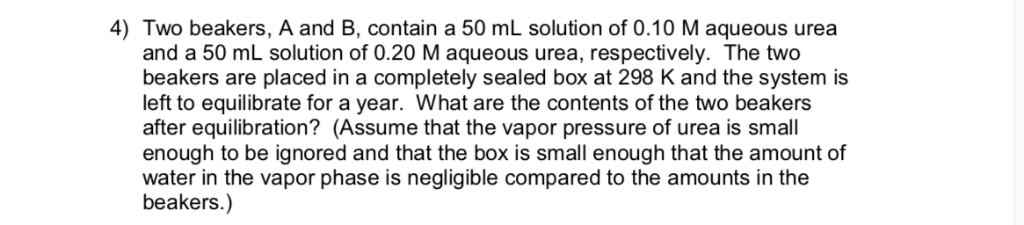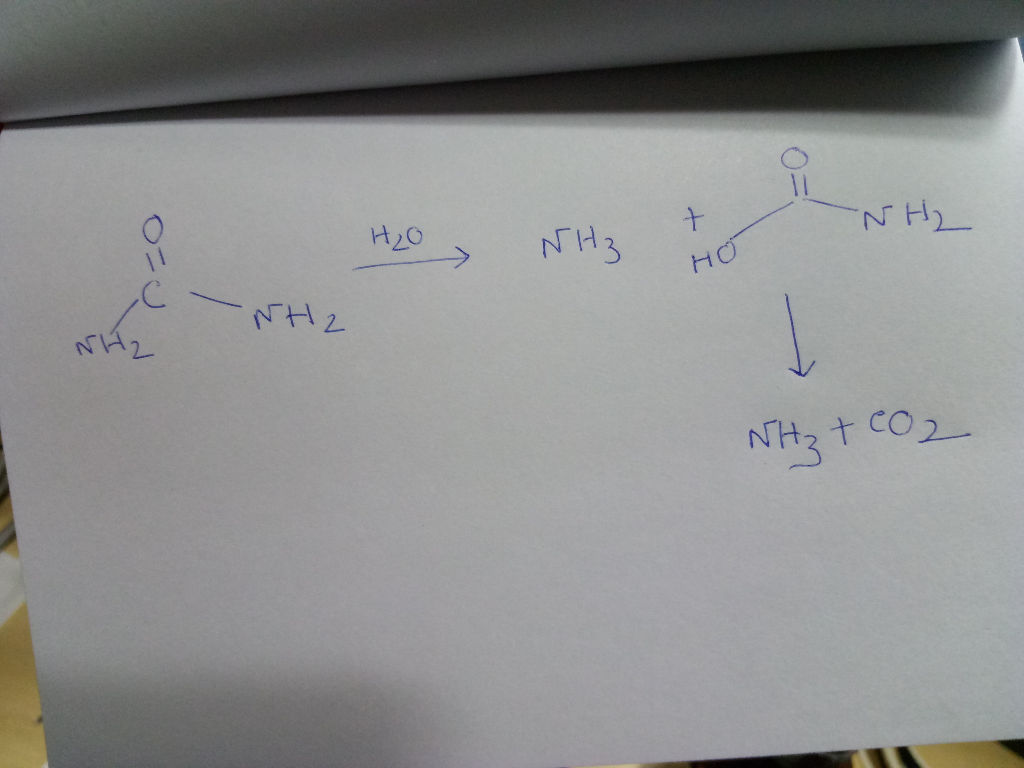Homework Help Question & Answers

# 4) Two beakers, A and B, contain a 50 mL solution of 0.10 M aqueous urea and a 50 mL solution of ...4) Two beakers, A and B, contain a 50 mL solution of 0.10 M aqueous urea and a 50 mL solution of 0.20 M aqueous urea, respectively. The two beakers are placed in a completely sealed box at 298 K and the system is left to equilibrate for a year. What are the contents of the two beakers after equilibration? (Assume that the vapor pressure of urea is small enough to be ignored and that the box is small enough that the amount of water in the vapor phase is negligible compared to the amounts in the beakers.)

#### Homework Answers

ReportAnswer #1

There will be no change in the concentration and contents of the two beakers after equilibration. It is mentioned that there is negligible amount of water in vapour phase so there will be no chance of hydrolysis of urea solution in the beakers. If there is some water contents then urea will convert into ammonia and carbon dioxide. But as mentioned that there is no water content there will be no change in the contents of this solutions.Add Homework Help Answer
##### Add Answer of: 4) Two beakers, A and B, contain a 50 mL solution of 0.10 M aqueous urea and a 50 mL solution of ...
Your Answer: Your Name: What's your source?
Not the answer you're looking for? Ask your own homework help question. Our experts will answer your question WITHIN MINUTES for Free.
More Homework Help Questions Additional questions in this topic.

• #### Two identical beakers are both half filled with a liquid

Need Online Homework Help?

Get FREE EXPERT Answers
WITHIN MINUTES
Related Questions